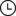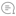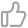# Python 3.8 一周后发布,这几个特性值得关注恋习Python |1062019-10-0900010月1日，Python 3.8rc1 发布，如果没有意外的话 3.8 将于 10 月 14 日正式发布。新版本的变化有很多，但是我觉得可能最常被用到的，是下面这两个新特性：海象运算符和仅位置参数。

## 海象运算符 :=

1. `m = re.match(p1, line)`

2. `if m:`

3. `return m.group(1)`

4. `else:`

5. ` m = re.match(p2, line)`

6. `if m:`

7. `return m.group(2)`

8. `else:`

9. ` m = re.match(p3, line)`

10. ` ...`

1. `if m := re.match(p1, line):`

2. `return m.group(1)`

3. `elif m := re.match(p2, line):`

4. `return m.group(2)`

5. `elif m := re.match(p3, line):`

6. ` ...`

1. `ent = obj.next_entry()`

2. `while ent:`

3. ` ... # process ent`

4. `ent = obj.next_entry()`

1. `while ent := obj.next_entry():`

2. ` ... # process ent`

## 使用 f-string 调试

Python 3.6 中就加入了 f-string（也被称为格式化字符串），但是在调试输出时的代码写法会显得比较重复：

1. `print(f'foo={foo} bar={bar}')`

1. `print(f'{foo=} {bar=}')`

1. `>>> import datetime`

2. `>>> now = datetime.datetime.now()`

3. `>>> print(f'{now=} {now=!s}')`

4. `now=datetime.datetime(2019, 7, 16, 16, 58, 0, 680222) now=2019-07-1616:58:00.680222`

## 仅位置参数（position-only）

1. `def f(a, b, /, c, d, *, e, f):`

2. `print(a, b, c, d, e, f)`

1. `f(10, 20, 30, d=40, e=50, f=60)`

1. `f(10, b=20, c=30, d=40, e=50, f=60) # b 不可以是关键字参数`

2. `f(10, 20, 30, 40, 50, f=60) # e 必须是关键字参数`

1. `def pow(x, y, z=None, /):`

2. `"Emulate the built in pow() function"`

3. ` r = x ** y`

4. `return r if z isNoneelse r%z`

1. `len(obj='hello') # obj 关键字降低了可读性`

1. `def quantiles(dist, /, *, n=4, method='exclusive')`

2. ` ...`

1. `>>>`

2. `>>> def f(a, b, /, **kwargs):`

3. `... print(a, b, kwargs)`

4. `...`

5. `>>> f(10, 20, a=1, b=2, c=3) # a 和 b 有两种用法`

6. `1020 {'a': 1, 'b': 2, 'c': 3}`

1. `classCounter(dict):`

2. `def __init__(self, iterable=None, /, **kwds):`

3. `# Note "iterable" is a possible keyword argument`

• https://lwn.net/Articles/793818/

• https://docs.python.org/3.8/whatsnew/3.8.html00

暂无评论~~
Ctrl+Enter